# MHD Mixed Convection Chemically Reactive Casson Fluid Flow over an Inclined Stretching/Shrinking Sheet: Paired Quasilinearization Approach (PQLM)

MHD Mixed Convection Chemically Reactive Casson Fluid Flow over an Inclined Stretching/Shrinking Sheet: Paired Quasilinearization Approach (PQLM)
Department of Mathematics, University of Swaziland, Swaziland

School of Mathematics, Statistics and Computer Science, University of KwaZulu-Natal, South Africa

Corresponding Author Email:
mekk_aya@yahoo.com
Page:
188-196
|
DOI:
https://doi.org/10.18280/mmep.060206
25 January 2019
|
Accepted:
4 June 2019
|
Published:
20 June 2019
| Citation

OPEN ACCESS

Abstract:

The aim of this paper is to investigate the flow, heat, and mass transfer qualities in a Casson liquid overflow over a stretching/shrinking sheet in the presence of transverse magnetic field by considering diffusion-thermal, thermo-diffusion, chemical reaction, and hall effects. This investigation is carried out by studying the influence of selected governing parameters, namely, chemical reaction, Hall, suction/blowing, and Soret and Dufour number on the respective flow profiles of velocity, temperature, and concentration and their corresponding effect on the skin-friction coefficient, local Nusselt number, and Sherwood number. The nonlinear governing equations are transformed into nonlinear partial differential equations and solved with the efficient paired quasilinearization method that seeks to decouple a large system of equations into coupled pairs of equations by linearizing two functions and their corresponding derivatives at a time. The study reveals that increasing the value of chemical reaction, Hall, Dufour and Soret parameters significantly impacted on the flow profiles. The results suggest that while increasing chemical reaction and Dufour number decreases velocity and concentration of the liquid, the Hall parameter increases them while decreasing the secondary velocity and temperature. Applications of the study arise in magnetic field control of materials processing systems, electric transformers, manufacture processes in plastic and polymer etc.

Keywords:

heat transfer, mass transfer, hydromagnetic flow, secondary flow, numerical solution, hall effect, chemical reaction, Soret and Dufour

1. Introduction

Mixed convective heat and mass transfer phenomena in the presence of magnetic fields arise in industrial and technological applications. Magnetohydrodynamic (MHD) flows have received wide attention from many researchers due to the significance of such flows which are known to be applicable in many devices such as accelerators, magnetohydrodynamic (MHD) pumps, MHD power generators, electrostatic precipitation, petroleum industry, purification of crude oil, aerodynamics heating and fluid droplets sprays. For the applications of hydromagnetics, however, the current trend is towards a strong magnetic field to notice the influence of an electromagnetic force. The Hall current is important under these conditions and has a marked effect on the magnitude and direction of the current density and consequently on the magnetic force term. Various researchers [1-8] have examined the channel flows of a viscous fluid under the action of a transversely applied magnetic field. The role of combined effect of magnetic field is significant in increasing or decreasing fluid velocity or skin-friction and also in attainment of steady state Oni . The influence of a magnetic field reduces both wall heat and mass transfer rates on the Hydromagnetic nanofluid flow due to a stretching or shrinking sheet Kameswaran et al. .

When heat and mass transfer occur simultaneously between the fluxes, the driving potential is of more intricate nature, as energy flux can be generated not only by temperature gradients but by composition gradients as well.

The energy flux caused by the concentration gradient and temperature gradient are called the Dufour (diffusion-thermal) effect and Soret (thermo-diffusion) effect, respectively. These effects should be taken into consideration when there is a density difference in the flow domain, for instance, both Dufour and Soret effects can be created when species are introduced at a surface in fluid domain, with a different density than the surrounding fluid. Dursunkaya and Worek  studied diffusion-thermo and thermal-diffusion effects in transient and steady natural convection vertical surface.  It is show in this study the dimensionless temperature ratio is decreased, the nondimensional wall heat flux shows a larger dependence on the Grashof number ratio.

Ahmed  studied the effect of both Dufour and Soret on free convective heat and mass transfer over a stretching surface with suction and injection using scaling transformations for similarity solutions to obtain a numerical solution. In this study, they showed that for fluids with medium molecular weight, (H2, air), there exists significant effects of Dufour and Soret. Turkyilmazoglu  analyzed magnetohydrodynamic fluid flow and heat transfer due to two-three dimensional porous and deforming (stretching/shrinking) bodies. The influence of thermal radiation, Soret and Dufour impacts, heat generation or absorption and substance response on blended convection stream over a vertical extending sheet in a permeable medium with suction/infusion was examined by Pal and Mondal . Mathematical modelling for heat transfer of a micropolar fluid along a permeable stretching/shrinking wedge with heat generation/absorption was studied by Alam et al . The results show that dimensionless temperature increases when the shrinking parameter is increased and decreases with the increase of stretching parameter, unsteadiness parameter and suction/injection parameter.

From the review above and to the knowledge of the authors, the combined convection heat transfer considering various parameters have not been examined. The present work focuses on the combined Soret and Dufour effects in MHD two-dimensional chemically reactive flow over stretching/shrinking sheet with suction/injection and Hall effect. The modeled partial differential equations are solved by a recently developed efficient numerical method named the paired quasilinearization method (PQLM) .

The remainder of this paper is organized as follows: Section 2 introduces the physical modelling of the problem and formulation of the governing equation, Section 3 describes the numerical scheme, Section 4 represents the results and discussion and section 5 presents conclusion.

2. Mathematical Model

Consider the steady-state boundary layer flow over a stretching/shrinking sheet in the presence of transverse magnetic field, diffusion-thermal, thermo-diffusion, and chemical reaction effects as displayed in Figure 1. The plate is inclined from the vertical plane with an acute angle γ and considered to be permeable (porous) to allow for possible fluid suction or blowing. It is also assumed that a strong magnetic field is perpendicular to the flow and the Hall effect (induced flow in the z- direction) is considered. To simplify the analysis, we assume that there is no variation of flow and heat transfer quantities in z-direction, which is valid if the plate would be of infinite width in this direction. The sheet has constant linear velocity uw(x)=cxp (for stretching sheet) and uw(x)=-cxa (for shrinking sheet).

## 1.jpg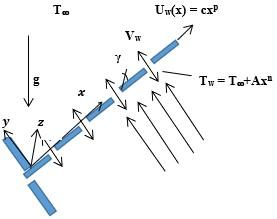Figure 1. Flow geometry

The rheological equation of state for an isotropic and incompressible Casson fluid is given by Prasad et al. :

{{\tau }_{ij}}\left\{ \begin{align} & 2\left( {{\mu }_{B}}+{{{P}_{y}}}/{\sqrt{2\pi }}\; \right){{e}_{ij}}\text{ }\pi >{{\pi }_{c}} \\ & 2\left( {{\mu }_{B}}+{{{P}_{y}}}/{\sqrt{2{{\pi }_{c}}}}\; \right){{e}_{ij}}\text{ }\pi <{{\pi }_{c}} \\ \end{align} \right.                      (1)

Based on Boussinesq approximations, the equations governing the steady-state conservation of mass, momentum,

energy and concentration with the assumptions above are given by

$\frac{\partial u}{\partial x}+\frac{\partial v}{\partial y}=0$                            (2)

$u\frac{\partial u}{\partial x}+v\frac{\partial v}{\partial y}=v\left[ \left| 1+\frac{1}{\beta } \right| \right]\frac{{{\partial }^{2}}u}{\partial {{y}^{2}}}+{{g}^{*}}\left[ {{\beta }_{T}}\left( T-{{T}_{\infty }} \right)+{{\beta }_{c}}\left( C-{{C}_{\infty }} \right) \right]\cos \gamma -\frac{\sigma B_{0}^{2}}{\rho \left( 1+{{m}^{2}} \right)}\left( u+mw \right)$                         (3)

$u\frac{\partial w}{\partial x}+v\frac{\partial w}{\partial y}=v\left[ \left| 1+\frac{1}{\beta } \right| \right]\frac{{{\partial }^{2}}w}{\partial {{y}^{2}}}+\frac{\sigma B_{0}^{2}}{\rho \left( 1+{{m}^{2}} \right)}\left( mu-w \right)$                     (4)

$u\frac{\partial T}{\partial x}+v\frac{\partial T}{\partial y}=\alpha \frac{{{\partial }^{2}}T}{\partial {{y}^{2}}}+\frac{D{{K}_{T}}}{{{C}_{s}}{{C}_{p}}}\text{ }\frac{{{\partial }^{2}}C}{\partial {{y}^{2}}}$                          (5)

$u\frac{\partial C}{\partial x}+v\frac{\partial C}{\partial y}=D\frac{{{\partial }^{2}}C}{\partial {{y}^{2}}}-{{K}_{1}}\left( C-{{C}_{\infty }} \right)+\frac{D{{K}_{T}}}{{{T}_{m}}}\text{ }\frac{{{\partial }^{2}}T}{\partial {{y}^{2}}}$                      (6)

The appropriate boundary conditions on velocities, temperature and concentration field are as follows:

$u_{w}=c x^{p}, \quad v=V_{w}, \quad w=0, \quad T=T_{\infty}=T_{\infty}+A x^{n}$,

$C=C_{w}=C_{\infty}+B x^{q}, \quad$ at $\quad y=0$                           (7a)

$u \rightarrow 0, \quad w \rightarrow 0, \quad T \rightarrow T_{\infty}, \quad C \rightarrow C_{\infty}$ as $\quad y \rightarrow \infty$                          (7b)

Vw is the wall mass flux with Vw < 0 for suction and Vw > 0 fluid blowing at the sheet surface.

We seek similarity solutions to the Eq. (3) to Eq. (6) with boundary conditions (7) in the following form:

$\xi=G r_{x} R e_{x}^{-2} \cos \gamma, \quad \eta=\frac{y}{x} R e_{x}^{1 / 2}, \quad w=\frac{y}{x} \operatorname{Re}_{x} g(\xi, \eta)$

$\psi=v R e_{x}^{-1 / 2} f(\xi, \eta), \quad \theta(\xi, \eta)=\frac{T-T_{\infty}}{T_{w}-T_{\infty}}, \quad \phi(\xi, \eta)=\frac{c-c_{\infty}}{C_{w}-C_{\infty}}$                             (8)

where stream function $\psi$ is defined as $u=\frac{\partial \psi}{\partial y}$ and $v=-\frac{\partial \psi}{\partial x}$ which identically satisfies the Eq. (2). We deduce from Eq. (8) that:

$u=\frac{v}{x} R e_{x} f^{\prime}(\xi, \eta)$,

$v=-\sqrt{\operatorname{cv} x^{p}}\left[\frac{p+1}{2} f-\xi(n-2 p+1) \frac{\partial f}{\partial \xi}+\frac{p-1}{2} \eta f^{\prime}\right]$

Substituting Eq. (8) into Eq. (2) to Eq. (6), we get the following equations:

$\left(1+\frac{1}{\beta}\right) f^{\prime \prime \prime}-p f^{\prime 2}+\frac{p+1}{2} f f^{\prime \prime}+\xi(\theta+\delta \phi)-\frac{M}{1+m^{2}}\left(f^{\prime}+m g\right)=\xi(n-2 p+1)\left(f^{\prime} \frac{\partial f^{\prime}}{\partial \xi}-f^{\prime \prime} \frac{\partial f}{\partial \xi}\right)$                               (9)

$\left(1+\frac{1}{\beta}\right) g^{\prime \prime}-p g f+\frac{p+1}{2} f g^{\prime}+\frac{M}{1+m^{2}}\left(m f^{\prime}-g\right)=\xi(n-2 p+1)\left(f^{\prime} \frac{\partial g}{\partial \xi}-g^{\prime} \frac{\partial f}{\partial \xi}\right)$                              (10)

$\frac{1}{P r} \theta^{\prime \prime}+\frac{p+1}{2} f \theta^{\prime}-n \theta f^{\prime}+D_{f} \phi^{\prime \prime}=\xi\left(f^{\prime} \frac{\partial \theta}{\partial \xi}-\theta^{\prime} \frac{\partial f}{\partial \xi}\right)$                            (11)

$\phi^{\prime \prime}+S c \frac{p+1}{2} f \phi^{\prime}-S c q \phi f^{\prime}+S c S t \theta^{\prime \prime}-S c K \phi=S c \xi\left(f^{\prime} \frac{\partial \phi}{\partial \xi}-\phi^{\prime} \frac{\partial f}{\partial \xi}\right)$                    (12)

where the prime denotes differentiation with respect to $\eta$. The boundary conditions now take the form:

$f(\xi, 0)=\frac{2}{p+1} f_{w}, \quad f^{\prime}(\xi, 0)=1, \quad g(\xi, 0)=0$,

$\theta(\xi, \eta)=1, \quad \phi(\xi, \eta)=1$                             (13a)

$f^{\prime} \rightarrow 0, \quad g \rightarrow 0, \quad \theta \rightarrow 0, \quad \phi \rightarrow 0 \quad$ as $\quad \eta \rightarrow 0$                     (13b)

where

$f_{w}=-\frac{x V_{w} R e_{x}^{-\frac{1}{2}}}{v}, \quad R e_{x}=\frac{u_{w}(x) x}{v}, \quad M=\frac{\sigma B_{0}^{2}}{\rho u_{w}}$

$G r_{x}=g^{*} \beta\left(T_{w}(x)-T_{\infty}\right) x^{3} / v, D f=\frac{D K_{T} B x^{q}}{c_{p} v\left(T_{w}-T_{\infty}\right)}$

$S t=\frac{D K_{T}\left(T_{w}-T_{\infty}\right)}{v T_{m}\left(C_{w}-C_{\infty}\right)}$

The nondimensional local shear stress components, Nusselt number and Sherwood number are given by:

$\begin{array}{ll}{\tau_{w x}=-\frac{\mu u_{w}}{x} R e_{x}^{\frac{1}{2}} f^{\prime \prime}(\xi, 0),} & {\tau_{w z}=-\frac{\mu u_{w}}{x} R e_{x}^{\frac{1}{2}} g^{\prime}(\xi, 0)} \\ {N u_{x} R e_{x}^{-\frac{1}{2}}=-\theta^{\prime}(\xi, 0),} & {S h_{x} R e_{x}^{-\frac{1}{2}}=-\phi^{\prime}(\xi, 0)}\end{array}$

3. Numerical Scheme

To solve the nonlinear system of partial differential Eq. (9) to Eq. (12), we apply the method of decoupling large systems into coupled pairs of equations called the ''Paired Quasilinearisation Method'' (PQLM) [14, 15]. The underlying idea of the PQLM is the quasilinearization method, introduced by Bellman and Kallaba , which works by linearizing a nonlinear system of equations using the Taylor series approach and discretising the linearized pairs of equations using spectral method. We elect to apply quasilinearization on the nonlinear functions f, g, and their corresponding derivatives and we obtain the linearized pair of equations.

$\left(1+\frac{1}{\beta}\right) f_{r+1}^{\prime \prime \prime}+\left[a_{1}\right] f_{r+1}^{\prime \prime}++\left[a_{2}\right] f_{r+1}^{\prime}+\left[a_{3}\right] f_{r+1}+(-2 m) g_{r+1}=\left[a_{4}\right] \frac{\partial f_{r+1}^{\prime}}{\partial \xi}+\left[a_{5}\right] \frac{\partial f_{r+1}}{\partial \xi}+a_{6}\left[b_{1}\right] f_{r+1}^{\prime}+\left[b_{2}\right] f_{r+1}+\left(1+\frac{1}{\beta}\right) g_{r+1}^{\prime \prime}+\left[b_{3}\right] g_{r+1}^{\prime}+\left[b_{4}\right] g_{r+1}=\left[b_{5}\right] \frac{\partial f_{r+1}}{\partial \xi}+\left[b_{6}\right] \frac{\partial g_{r+1}}{\partial \xi}+\left[b_{7}\right]$  (14)

where

$\alpha_{1}=\frac{p+1}{2}, \quad \alpha_{2}=\frac{M}{1+m^{2}}, \quad \alpha_{3}=n-2 p+1$                                 (15)

$a_{1}=\alpha_{1} f_{r}+\xi \alpha_{3} \frac{\partial f_{r}}{\partial \xi}, a_{2}=-2 p f_{r}^{\prime}-\alpha_{2}-\xi \alpha_{3} \frac{\partial f_{r}^{\prime}}{\partial \xi}$                                     (16)

$a_{1}=\alpha_{1} f_{r}^{\prime \prime}, \quad a_{4}=\alpha_{1} \xi \alpha_{3} f_{r}^{\prime}, \quad a_{5}-\alpha_{3} \xi \alpha_{3} f_{r}^{\prime \prime}$                                 (17)

$a_{6}=-p f_{r}^{\prime 2}+\frac{p+1}{2} f_{r} f_{r}^{\prime \prime}-\xi\left(\theta_{r}+\delta \phi_{r}\right)-\xi \alpha_{3} f_{r}^{\prime} \frac{\partial f_{r}^{\prime}}{\partial \xi}+\xi \alpha_{3} f_{r}^{\prime} \frac{\partial f_{r}}{\partial \xi}$                                  (18)

$b_{1}=-p g_{r}+\alpha_{2} m-\xi \alpha_{3} \frac{\partial g_{r}}{\partial \xi}, \quad b_{2}=\alpha_{1} g_{r}^{\prime}$                                      (19)

$b_{3}=\frac{p+1}{2} f_{r}+\xi \alpha_{3} \frac{\partial f_{r}}{\partial \xi}, \quad b_{4}=-p f_{r}^{\prime}-\alpha_{2}$                                  (20)

$b_{5}=\xi \alpha_{3} g_{r}^{\prime}, \quad b_{6}=\xi \alpha_{3} f_{r}^{\prime}, b_{7}=p f_{r}^{\prime} g_{r}+\alpha_{1} f_{r} g_{r}^{\prime}-\xi \alpha_{3}\left(f_{r}^{\prime} \frac{\partial g_{r}}{\partial \xi}-g_{r}^{\prime} \frac{\partial f_{r}}{\partial \xi}\right)$                              (21)

where […] are vector representations and terms with r+1 and r subscripts are at current and previous iteration levels, respectively. Updated solutions for f, g, and their corresponding derivatives are used in the subsequent pair of equations which is linear and we obtain:

$\frac{1}{\operatorname{Pr}} \theta_{r+1}^{\prime \prime}+\left[c_{1}\right] \theta_{r+1}^{\prime}+c_{2} \theta_{r+1}+D f \phi_{r+1}^{\prime \prime}=\left[c_{3}\right] \frac{\partial \theta_{r+1}}{\partial \xi}$

$S c \operatorname{Sr} \theta_{r+1}^{\prime \prime}+\phi_{r+1}^{\prime \prime}+\left[e_{1}\right] \phi_{r+1}^{\prime}+e_{2} \phi_{r+1}=\left[e_{3}\right] \frac{\partial \phi_{r+1}}{\partial \xi}$                                      (22)

where

$e_{1}=S c\left(\frac{p+1}{2} f_{r}, \xi \frac{\partial f_{r}}{\partial \xi}\right), e_{2}=-S c\left(q f_{r}^{\prime}+K\right), e_{2}=S c \xi f_{r}^{\prime}$                             (23)

The linearized pairs of Eq. (14) and Eq. (22) are now solved using the bivariate spectral collocation method with Chebyshev-Gauss-Lobatto nodes. Before discretizing the spacial η and time ξ domains, we first transform the original semi-infinite domains using linear transformations from $\eta \in[0, \infty)$ and $\xi \in[0, \infty)$ to $x \in[-1,1]$ and $t \in[-1,1]$. $\eta_{\infty}$ and $\xi_{\infty}$ are finite values that are appropriately chosen to be large values that properly approximates the characteristics of the flow quantities close to infinity. It is necessary to introduce these values to apply the spectral method at the infinity boundary. We define the discretization nodes as:

$x_{i}=\cos \left(\frac{\pi i}{M_{x}}\right), \quad t_{j}=\cos \left(\frac{\pi j}{M_{t}}\right), \quad i=1,2, \ldots, M_{x} \quad j=1,2, \ldots, M_{t}$                                 (24)

An assumption is made that the approximate solutions are defined using the bivariate Lagrange interpolation polynomial of the form:

$E(\eta \xi) \approx \sum_{m=0}^{M_{x}} \sum_{j=0}^{M_{t}} E\left(x_{m}, t_{j}\right) L_{j}(t), \quad E=f, g, \theta, \phi$                                  (25)

that interpolates $E(\eta \xi)$ at the Gauss-Lobatto collocation points. The Chebyshev pseudo-spectral method is applied on the pairs Eq. (14) and Eq. (22) with derivatives of the unknown functions with respect to $\eta$ and $\xi$ at the collocation points $x_{k}$ and $t_{i}$ defined as:

$\left.\frac{\partial^{n} E}{\partial \eta}\right|_{\left(x_{k} t_{i}\right)}=\boldsymbol{D}^{n} \boldsymbol{E}_{i}, \quad n=1,2,3$                               (26)

$\left.\frac{\partial E}{\partial \eta}\right|_{\left(x_{k} t_{i}\right)}=\sum_{j=0}^{M_{t}} \boldsymbol{d}_{i j} \boldsymbol{E}_{j}$                              (27)

where $\boldsymbol{d}_{i j}=\left(\frac{2}{x_{e}}\right) d_{i j} \quad\left(i, j=0,1, \ldots, M_{t}\right)$ with $d_{i j}$ being entries of the standard Chebyshev differentiation matrix $d=\left[d_{i j}\right]$ of size $\left(M_{t}+1\right) X\left(M_{t}+1\right)$ (see, for example Trefethen ). Similarly, $D=\left(\frac{2}{\eta_{c}}\right)\left[D_{r, s}\right]\left(r, s=0,12, \ldots, M_{t}\right)$ with $\left[D_{r, s}\right]$ being an $\left(M_{x}+1\right) X\left(M_{x}+1\right)$ Chebyshev derivative matrix, and the vector $E_{i}$ is defined as:

$\boldsymbol{E}_{i}=\left[E_{i}\left(x_{0}\right), E_{i}\left(x_{1}\right), \ldots, E_{i}\left(x_{M x}\right)\right]^{T}$                                (28)

The discretized forms of the pairs of Eq. (14) and Eq. (22) are:

$A_{1,1}^{(i)} \boldsymbol{F}_{r+1, i}+\boldsymbol{A}_{1,2}^{(i)} \boldsymbol{G}_{r+1, i}+a_{5} \sum_{j=0}^{M_{t}} \boldsymbol{d}_{i j} \boldsymbol{F}_{r+1}, j+a_{4} \sum_{j=0}^{M_{t}} \boldsymbol{d}_{i j} \boldsymbol{D} \boldsymbol{F}_{r+1, j}=\boldsymbol{R}_{1, i}$                         (29)

$A_{2,1}^{(i)} F_{r+1, i}+A_{2,2}^{(i)} G_{r+1, i}+b_{5} \sum_{j=0}^{M_{t}} d_{i j} F_{r+1, j}+b_{6} \sum_{j=0}^{M_{t}} d_{i j} G_{r+1, j}=R_{2, i}$                           (30)

$\boldsymbol{A}_{3,3}^{(i)} \boldsymbol{\theta}_{r+1, i}+c_{4} \sum_{j=0}^{M_{t}} \boldsymbol{d}_{i j} \boldsymbol{\theta}_{r+1, j}=\boldsymbol{R}_{3, i}$                      (31)

$\boldsymbol{A}_{4,4}^{(i)} \Phi_{r+1, i}+e_{2} \sum_{j=0}^{M_{t}} \boldsymbol{d}_{i j} \Phi_{r+1, j}=\boldsymbol{R}_{4, i}$                     (32)

for $i=0,1, \ldots, M_{t}$ where the matrix coefficients and right hand side vectors are defined as:

$\boldsymbol{A}_{1,1}^{(i)}=\left(1+\frac{1}{\beta}\right) \boldsymbol{D}^{3}+\left[a_{1}\right] \boldsymbol{D}^{3}+\left[a_{2}\right] \boldsymbol{D}+\left[a_{3}\right]-\left[a_{4}\right] \boldsymbol{d}_{i, j}-\left[a_{5}\right] \boldsymbol{d}_{i, j}$  (33)

$\boldsymbol{A}_{1,2}^{(i)}=-\alpha_{2} m \boldsymbol{I}, \boldsymbol{A}_{2,1}^{(i)}=\left[b_{1}\right] \boldsymbol{D}+\left[\boldsymbol{b}_{2}\right]-\left[b_{5}\right] \boldsymbol{d}_{i, j}$                             (34)

$\boldsymbol{A}_{2,2}^{(i)}=\left(1+\frac{1}{\beta}\right) \boldsymbol{D}^{2}+\left[b_{3}\right] \boldsymbol{D}+\left[b_{4}\right]-\left[b_{6}\right] \boldsymbol{d}_{i j}$                             (35)

$\boldsymbol{A}_{3,3}^{(i)}=\frac{1}{\operatorname{Pr}} \boldsymbol{D}^{2}+\left[c_{1}\right] \boldsymbol{D}+\left[c_{2}\right]-\left[c_{3}\right] \boldsymbol{d}_{i, j}$                             (36)

$\boldsymbol{A}_{4,4}^{(i)}=\boldsymbol{D}^{2}+\left[e_{1}\right] \boldsymbol{D}+\left[e_{2}\right]-\left[e_{3}\right] \boldsymbol{d}_{i, j}$                                 (37)

$A_{3,4}^{(i)}=D f D^{2}, \quad A_{4,3}^{(i)}=S c S r D^{2}$                               (38)

$\boldsymbol{R}_{1, i}=\left[a_{4}\right] \boldsymbol{d}_{i, M_{i}+1} \boldsymbol{D} \boldsymbol{F}_{i, M_{i}+1}+\left[a_{5}\right] \boldsymbol{d}_{i, M_{i}+1} \boldsymbol{F}_{i, M_{i}+1}+a_{6}$                                  (39)

$\boldsymbol{R}_{2, i}=\left[b_{5}\right] \boldsymbol{d}_{i, M_{i}+1} \boldsymbol{D} \boldsymbol{F}_{i, M_{i}+1}+\left[b_{6}\right] \boldsymbol{d}_{i, M_{i}+1} \boldsymbol{G}_{i, M_{i}+1}+b_{7}$                               (40)

$\boldsymbol{R}_{3, i}=\left[c_{3}\right] \boldsymbol{d}_{i, M_{i}+1} \boldsymbol{\theta}_{i, M_{i}+1}$                                     (41)

$\boldsymbol{R}_{4, i}=\left[e_{3}\right] \boldsymbol{d}_{i, M_{i}+1} \Phi_{i, M_{i}+1}$                                  (42)

where I is an identity matrix of size $\left(M_{x}+1\right) X\left(M_{x}+1\right)$.

4. Results and Discussion

We present the results generated for the system of partial differential Eq. (9) to Eq. (12) using the PQLM. We note that these results were generated using 80 grid points in space and 10 grid points in time as they were observed to be sufficient in obtaining convergent and accurate solutions. Convergence and accuracy were tested using solution error and residual error, respectively.

Figures 2a to 2d display the effect of iterations on the solution errors of the various functions f, g, θ and ϕ. These solution errors are obtained as the difference between successive iterations. We remark that these graphs were obtained when P = 0.1, n = 0.1, fw = -1, m = 0.5, df = 0.1, sr= 0.6, k = 4, $\delta$= 1, sc = 0.22, pr = 0.7, and $\boldsymbol{\beta}=\infty$.

We observe from Figures 2a and 2c that as the number of iterations increase, the error between successive functions gets smaller. By the 14th iteration, we see that the error is at 10-14 which implies that the difference between solutions of f and $\theta$ subsequently are negligible since the error is minimal. This indicates that the solutions can be said to converge if we set our tolerance level to be 10-14. We observe from Figures 2b and 2d that the difference between solutions for the functions g and $\phi$ remains relatively constant and very small implying that convergence occurs immediately we begin iterating. This indicates that our method is convergent for models similar to ours.

# 2a.jpg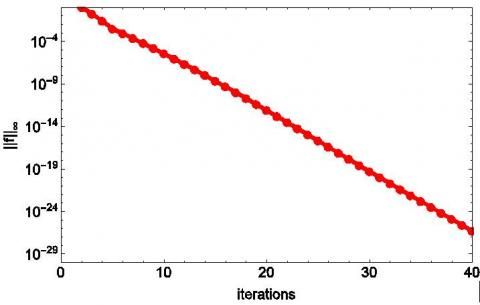(a) Effect of iterations on the solution error norm for F

## 2b.jpg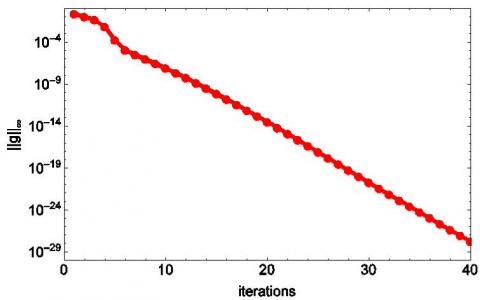(b) Effect of iterations on the solution error norm for G

## 2c.jpg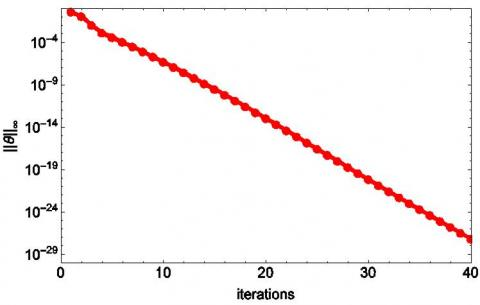(c) Effect of iterations on the solution error norm for $\Theta$

## 2d.jpg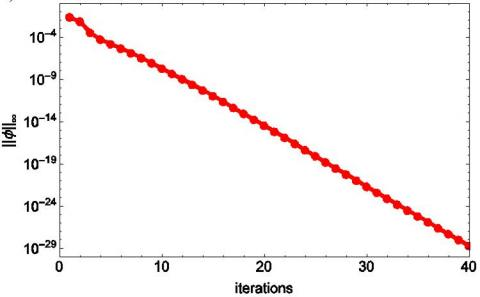(d) Effect of iterations on the solution error norm for Ф

Figure 2. Solution errors

To test for accuracy, we make use of the residual error. This is defined as the error obtained after representing the original system of differential equations with the approximate solutions produced using a numerical method. We define the residual errors as:

$\operatorname{Res}(\mathbf{F})=\max _{0 \leq i \leq M_{i}} \|\left(1+\frac{1}{\beta}\right) f_{i}^{\prime \prime \prime}-p f_{i}^{\prime 2}+\frac{p+1}{2} f_{i} f_{i}^{\prime \prime}+\xi\left(\theta_{i}+\phi_{i}\right)-\frac{M}{1+m^{2}}\left(f_{i}^{\prime}+m g_{i}\right)-\xi(n-2 p+1)\left(f_{i}^{\prime} \frac{\partial f_{i}^{\prime}}{\partial \xi}-f_{i}^{\prime} \frac{\partial f_{i}}{\partial \xi}\right) \|_{\infty}$                                (43)

$\operatorname{Res}(\mathbf{G})=\max _{0 \leq i \leq M_{i}} \|\left(1+\frac{1}{\beta}\right) g_{i}^{\prime \prime}-p g_{i} f_{i}^{\prime 2}+\frac{p+1}{2} f_{i} g_{i}^{\prime}+\frac{M}{1+m^{2}}\left(m f_{i}^{\prime}-g_{i}\right)-\xi(n-2 p+1)\left(f_{i}^{\prime} \frac{\partial g_{i}}{\partial \xi}-g_{i}^{\prime} \frac{\partial f_{i}}{\partial \xi}\right) \|_{\infty}$                                   (44)

$\operatorname{Res}(\Theta)=\max _{0 \leq i \leq M_{i}}\left\|\frac{1}{\operatorname{Pr}} \theta_{i}^{\prime \prime}+S c \frac{p+1}{2} f_{i} \theta_{i}^{\prime}-n f_{i}^{\prime} \theta_{i}+D f \phi_{i}^{\prime \prime}-\xi\left(f_{i}^{\prime} \frac{\partial \theta_{i}}{\partial \xi}-\theta_{i}^{\prime} \frac{\partial f_{i}}{\partial \xi}\right)\right\|_{\infty}$                                   (45)

$\operatorname{Res}(\mathbf{\Phi})=\max _{0 \leq i \leq M_{i}}\left\|\frac{1}{S c} \phi_{i}^{\prime \prime}+\frac{p+1}{2} f_{i} \phi_{i}^{\prime}-q \phi_{i} f_{i}^{\prime}-K \phi_{i}-\xi\left(f_{i}^{\prime} \frac{\partial \phi_{i}}{\partial \xi}+S t \theta_{i}-\phi_{i}^{\prime} \frac{\partial f_{i}}{\partial \xi}\right)\right\|_{\infty}$                                        (46)

From Figures 3a to 3d, we observe that the accuracy of the PQLM is relatively high as we observe that the error is smaller than 10-30 after 30 iterations for all the equations. We also observe that the Figure 2a does not attain convergence after 40 iterations. This is as a result of the number of different functions that are present in Eq. (9) hence the need to iterate further to attain convergence. Nonetheless, we observe that the error after the 30th iteration is sufficient to conclude that the PQLM is a highly accurate method for solving similar problems.

## 3a.jpg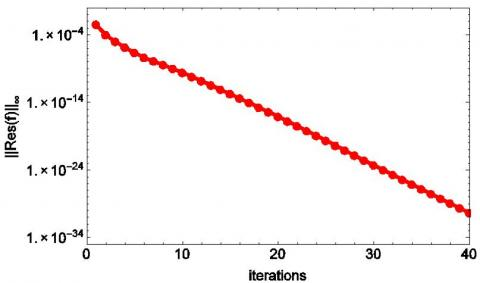(a) Effect of iterations on the residual error norm for F

## 3b.jpg(b) Effect of iterations on the residual error norm for G

## 3c.jpg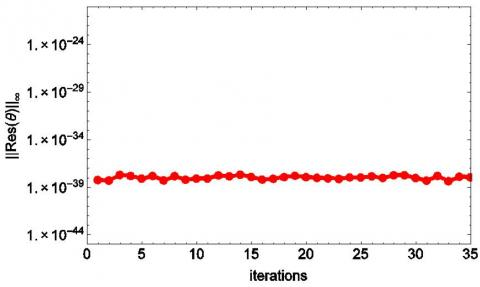(c) Effect of iterations on the residual error norm for $\Theta$

## 3d.jpg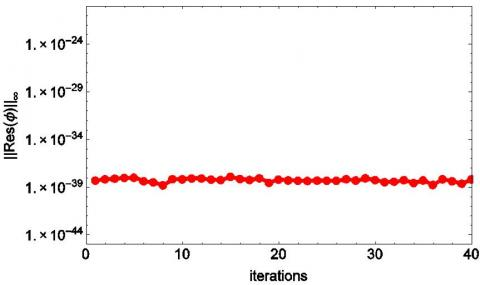(d) Effect of iterations on the residual error norm for Ф

Figure 3. Residual errors

The influence of some parameters on the various flow profiles are investigated using specific values of other parameters. The value of the Schmidt number (Sc) is chosen to be more realistic, 0.22 water, at 25 0C at 1 atmospheric pressure and Prandtl number Pr=0.7 throughout the study unless stated otherwise.

4.1 Skin friction

In this section, we present the skin friction, local Nusselt number and local Sherwood number at selected time levels. We begin by validating our results by comparing to results obtained using the bivariate spectral quasilinearization method (BSQLM). Table 1 displays the comparison of the skin friction obtained using the PQLM and the BSQLM when fw=1,  Df = 0.3, p = 0.1, $\beta$= $\infty$, M = 0.5, $\delta$= 1, n = 0.1, K = 4, q = 0.1, Pr = 0.7, St = 7.5, Sc = 0.22, and m = 2.

We observe from Table 1 that the results are in excellent agreement with those obtained using the BSQLM. This indicates that the PQLM produces the true approximate solutions to the problem under investigation.

Table 2 displays the effect of Dufour, Soret and chemical reaction on the skin friction, Nusselt number and Sherwood number.

Table 1. Comparison of skin friction -f''(ξ, 0) obtained using the BSQLM and PQLM at different time levels (ξ)

 ξ BSQLM PQLM 0.1 1.20896 1.208965 0.3 0.863117 0.863117 0.5 0.547564 0.547564 0.7 0.287002 0.287002 0.9 0.027817 0.027817

Table 2. Effect of parameters on f''(ξ,0), g'(ξ,0), $\theta^{\prime}$(ξ,0) and $\phi^{\prime}$(ξ,0)

 Df St K f''(ξ,0) g'(ξ,0) $\theta^{\prime}$(ξ,0) $\phi^{\prime}$(ξ,0) 0.01 6 1 0.337433 0.24442 -1.09904 0.326587 0.1 0.6 1 0.006082 0.21150 -1.02429 -0.58531 0.2 0.3 1 0.000193 0.21076 -0.98630 -0.63456 0.3 0.2 1 0.007583 0.21160 -0.95028 -0.65135 0.1 0.6 0 0.189086 0.25041 -1.06529 -0.31612 0.1 0.6 2 -0.07883 0.19752 -0.99988 -0.76640 0.1 0.6 3 -0.13320 0.18993 -0.98192 -0.91167 0.1 0.6 4 -0.17250 0.18511 -0.96754 -1.03614

From Table 2, we observe that while keeping chemical reaction constant, increasing the effect of Dufour and decreasing Soret leads to decrease of the skin friction and Sherwood number while increasing the Nusselt number. We also observe that when the value of Dufour gets larger than the value of Soret, skin friction also increases. As chemical reaction parameter increases, we observe that the skin friction and Sherwood number decreases while the Nusselt number increases.

4.2 Boundary layer distribution of velocities, temperature and concentration

4.2.1 With varying of chemical reaction parameter K

From Figures 4a to 4d, it is seen that both the primary and secondary flow decreases with increase in chemical reaction parameter. It is also observed that the temperature of the fluid increases due to increase in the chemical reaction while concentration of the fluid decreases. We also note that temperature decreases as chemical reaction increases when η>4 for blowing and η>6 for suction. We observe that the trends of the different profiles are similar regardless of the presence of suction or blowing. Figure 4c represents the temperature profiles for different values of K. In the neighborhood of the surface, the temperature profiles become maximum and then decrease and finally take asymptotic values. The decrease in the concentration profile in Figure 4d as the chemical reaction parameter is increased is due to the depletion of the chemical in the fluid.

## 4a.jpg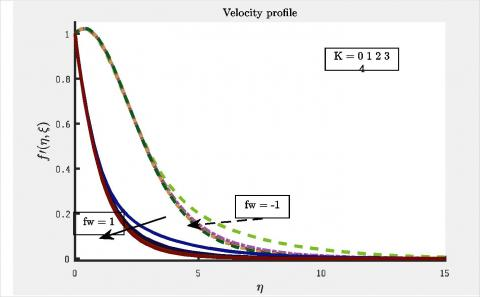(a) Effect of K on the velocity profile

## 4b.jpg(b) Effect of K on secondary velocity profile

## 4c.jpg(c) Effect of K on temperature profile

## 4d.jpg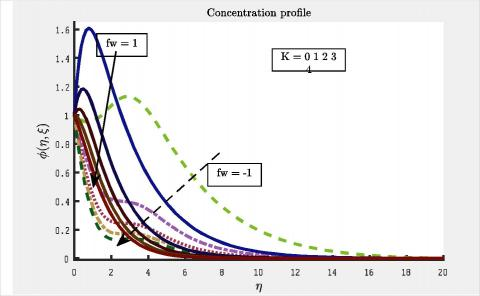(d) Effect of K on concentration profile

Figure 4. Effect of K

4.2.2 With varying of Dufour Df and Soret St numbers

Figures 5a to 5d display the combined influence of Dufour Df and Soret St on the velocity profiles, temperature profile and concentration profile. The variations are performed such that the differing values of Df and St give a constant value when multiplied. The specified values of the other parameters are p=0.1, $\beta=\infty$, M=0.5, $\delta$=1, n=0.1, K=4, q=0.1, Pr=0.7, Sc=0.22, and m=2.

The available studies on the Dufour and Soret effects show that Df and St are arbitrary constants, which provides that their product is constant. It is observed from Figures 5a and 5b that both primary and secondary velocities increase when there is an increase in the Dufour number and a corresponding decrease in the Soret number. An increase in Df causes a concentration gradient, and this concentration gradient plays an important role in the transportation of the heat energy from the solid boundary into the fluid, which results in an increase in the temperature as shown in Figure 5c. The effect of Dufour and Soret numbers on the concentration field is found in Figure 5d. Increasing the Dufour number (while decreasing the Soret number) leads to a decrease in the concentration boundary layer thickness.

## 5a.jpg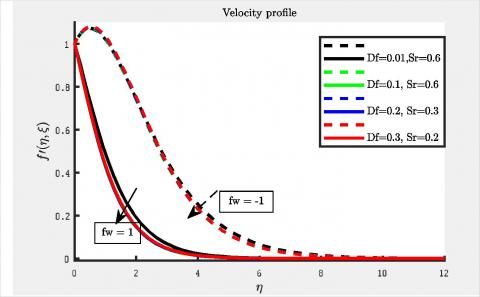(a) Effect of Df and St on the velocity profile

## 5b.jpg(b) Effect of Df and St on secondary velocity profile

## 5c.jpg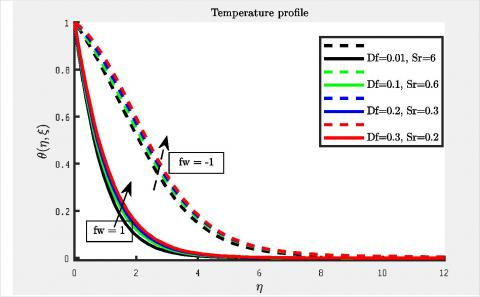(c) Effect of Df and St on temperature profile

## 5d.jpg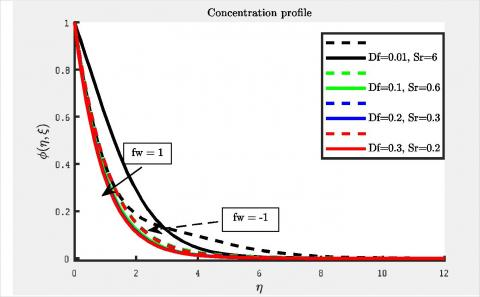(d) Effect of Df and St on concentration profile

Figure 5. Effect of K

4.2.3 With varying of Hall parameter m

Figures 6a to 6d display the effect of the Hall parameter m on the various profiles when p=0.1, $\beta=\infty$, M=0.5, $\delta$=1, n=0.1, Df=0.4, q=0.1, Pr=0.7, St=7.5, Sc=0.22, and K=4.

## 6a.jpg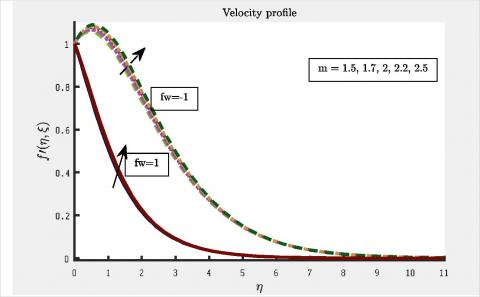(a) Effect of m on velocity profile

## 6b.jpg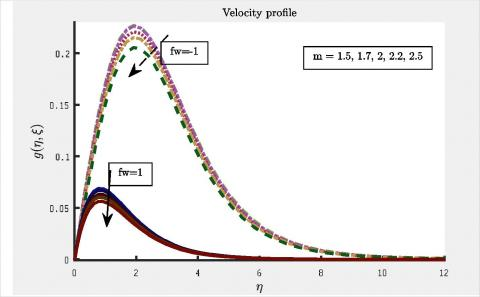(b) Effect of m on secondary velocity profile

## 6c.jpg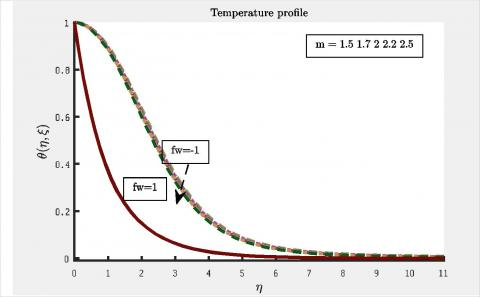(c) Effect of m on temperature profile

## 6d.jpg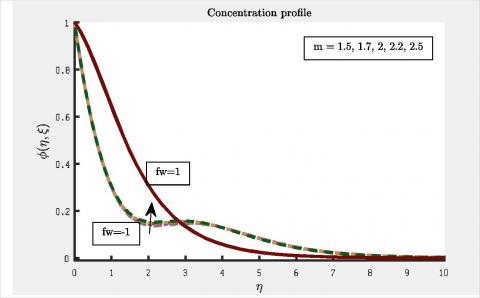(d) Effect of m on concentration profile

Figure 6. Effect of m

We observe that an increase in the Hall parameter increases the primary velocity but decreases the secondary velocity as shown in Figures 6a and 6d. In Figures 6c and 6d, we observe that blowing has no effect on the temperature and concentration profiles as the Hall parameter is increased. During suction, however, we observe that the temperature cools down while concentration increases when the Hall parameter is increased.

5. Conclusion

In this paper, investigation was conducted on the combined effect of Hall, suction/blowing parameter, chemical reaction parameters, Dufour and Soret number on flow over a stretching/shrinking sheet. The PQLM was used to obtain some useful results needed to illustrate the flow characteristics of the fluid and their dependence on some certain parameters.

• It was observed that increasing the chemical reaction parameter and Dufour number has a retarding effect on the velocity of flow field as well as concentration distributions.
• The hydrodynamic and concentration boundary layer thickness were observed to decrease as a result of increasing chemical reaction or Dufour effect.
• Velocity and temperature profiles with suction parameter (fw) were greater than velocity and temperature profiles of blowing parameter whereas the reverse effect is seen for concentration profile.
• Increasing the Hall parameter retards the secondary velocity and temperature profiles while enhancing the primary velocity and concentration profiles.
• The hydrodynamic and concentration boundary layer thickness were observed to decrease as a result of increasing chemical reaction.

Future studies will consider the porous medium flow and deception effects where the fluid is polar fluids (fluids with local rotary inertia and couple stresses). The governing equations will be solved by a new numerical approach and numerical simulations will be performed.

Nomenclature
 u, v, w Velocity components CP specific heat, J. kg-1. K-1 g* gravitational acceleration, m.s-2 Nu Sh T local Nusselt number along the heat source Sherwood number Temperature C n, p, q, A, B x, y, z D                 M Greek symbols Concentration are positive constants axes species diffusivity  magnetic parameter a thermal diffusivity, m2. s-1 bT thermal expansion coefficient, K-1 β Casson parameter f solid volume fraction Ɵ dimensionless temperature µ dynamic viscosity, kg. m-1.s-1 ρ $\beta_{C}$ fluid density coefficient of expansion with concentration Subscripts p nanoparticle f fluid (pure water) fw nanofluid Grx local Grashof number Df Dufour number St Soret number Rex Local Reynolds number B0 strength of the magnetic field Tm mean fluid temperature
References

 Chamkha AJ. (1995). Hydromagnetic two-phase flow in a channel. Int J Eng. Sci. 33: 437-46. https://doi.org/10.1016/0020-7225(93)E0006-Q

 Oni MO, Yusuf TS. (2017).  Unsteady couette flow in an annulus with combined mode of magnetic field application: A generalization. Mathematical Modelling of Engineering Problems 4(4): 168-172. https://doi.org/10.18280/mmep.040405

 Kameswaran PK, Narayana M, Sibanda P, Murthy PVSN. (2012). Hydromagnetic nanofluid flow due to a stretching or shrinking sheet with viscous dissipation and chemical reaction effects. Int. J. Heat Masstransf 55(25-26): 7587-7595. https://doi.org/10.1016/j.ijheatmasstransfer.2012.07.065

 Mansur S, Ishak A, Pop I. (2015). The magnetohydrodynamic stagnation point flow of nanofluid over a stretching/shrinking sheet with suction. PloSOne 10(3): e0117733. https://doi.org/10.1371/journal.pone.0117733

 Alam Md. S. (2016). Mathematical modelling for the effects of thermophoresis and heat generation/absorption on MHD convective flow along an inclined stretching sheet in the presence of Dufour-Soret effects. Mathematical modelling of Engineering problems 3(3): 119-128. https://10.18280/mmep.030302

 Sato H. (1961). The Hall effect in the viscous flow of ionized gas between parallel plates under transverse magnetic field. J Phys Soc Jpn 16(7): 1427-1435. https://doi.org/10.1143/JPSJ.16.1427

 Srinivasacharya D, Shiferaw M. (2009). Hydromagnetic effects on the flow of a micropolar fluid in a diverging channel. ZAMM Z. Angew. Math. Mech. 89(2): 123-131. https://doi.org/10.1002/zamm.200800035

 Abo-Eldahab Emad M, El-Aziz MA, Salem AM, Jaber KK. (2007).  Hall current effect on MHD mixed convection flow from an inclined continuously stretching surface with blowing/suction and internal heat generation/absorption. Applied Mathematical Modelling 31: 1829-1846. https://doi.org/10.1016/j.apm.2006.06.017

 Dursunkaya Z, Worek WM. (1992). Diffusion-thermo and thermal-diffusion effects in transient and steady natural convection from vertical surface. International Journal of Heat and Mass Transfer 35(8): 2060-2065. https://doi.org/10.1016/0017-9310(92)90208-A

 Ahmed AA. (2009). Similarity solution in MHD: Effects of thermal diffusion and diffusion thermo on free convective heat and mass transfer over a stretching surface considering suction or injection, Commun. Nonlinear Sci. Numer. Simul. 14: 2202. https://doi.org/10.1016/j.cnsns.2008.07.001

 Turkyilmazoglu M. (2016). Equivalence and corrspondance beteen the diforming body indueced flow and heat tow-three dimensions. Phys. Fluids 28: 043102. https://doi.org/10.1063/1.4945650

 Pal D, Mondal H. (2012). Influence of chemical reaction and thermal radiation on mixed convection heat and mass transfer over a stretching sheet in Darcian porous medium with Soret and Dufour effects. Energy. Convers. Manage 62: 102-108. https://doi/10.1016/j.enconman.2012.03.017

 Alam Md. S, Islam T, Uddin MJ. (2016). Mathematical modelling for heat transfer of a micropolar fluid along a permeable stretching/shrinking wedge with heat generation/absorption. Mathematical Modelling of Engineering Problems 3(1): 1-9. https://doi/10.18280/mmep.030101

 Motsa SS, Animasaun IL. (2016). Paired quasi-linearization analysis of heat transfer in unsteady mixed convection nanofluid containing both nanoparticles and gyrotactic microorganisms due to impulsive motion. J. Heat Transfer 138(11): 114503. https://doi/10.1115/1.4034039

 Otegbeye O, Motsa SS. (2018). A paired quasilinearization method for solving boundary layer flow problems. AIP Conference Proceedings 1975(1): 30020. https://doi.org/10.1063/1.5042190

 Bellman R, Kalaba R. (1965). Quasilinearization and Nonlinear Boundary Value Problems, Amer. Elseiver, New York.

 Trefethen LN. (2000). Spectral methods in MATLAB. SIAM, Oxford, England.